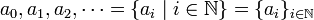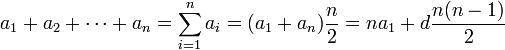# Arithmetic sequence

An arithmetic sequence (or arithmetic progression) is a (finite or infinite) sequence of (real or complex) numbers such that the difference of consecutive elements is the same for each pair.

Examples for arithmetic sequences are

• 2, 5, 8, 11, 14, 17 (finite, length 6: 6 elements, difference 3)
• 5, 1, −3, −7 (finite, length 4: 4 elements, difference −4)
• 1, 3, 5, 7, 9, ... (2n − 1), ... (infinite, difference 2)

## Mathematical notation

A finite sequence$a_1,a_2,\dots,a_n = \{ a_i \mid i=1,\dots,n \} = \{ a_i \}_{i=1,\dots,n}$

or an infinite sequence$a_0,a_1,a_2,\dots = \{ a_i \mid i\in\mathbb N \} = \{ a_i \}_{i\in\mathbb N}$

is called arithmetic sequence if

ai + 1ai = d

for all indices i. (The index set need not start with 0 or 1.)

### General form

Thus, the elements of an arithmetic sequence can be written as

ai = a1 + (i − 1)d

### Sum

The sum (of the elements) of a finite arithmetic sequence is$a_1 + a_2 +\cdots+ a_n = \sum_{i=1}^n a_i = (a_1 + a_n){n \over 2} = na_1 + d {n(n-1) \over 2}$Some content on this page may previously have appeared on Citizendium.